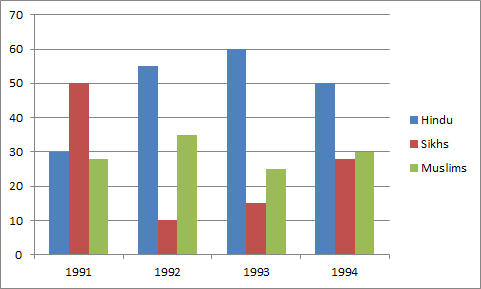# Data Interpretation - Bar Graph

>>>>>>>>Bar Graph

Instructions:

Given below is a bar diagram and percentage of Hindus, Sikhs, and Muslims in a state during the years from 1991-1994Study the above diagram and answer the following questions 21-25

• A

3 : 2• B

2 : 3• C

Cannot be calculated• D

None of these• Option : B
• Explanation : In 1991, the percentage of Hindus and Sikhs were 30 and 45 respectively. So, the ratio of Hindus and Sikhs was

30 : 45 or 2 : 3

Instructions:

Given below is a bar diagram and percentage of Hindus, Sikhs, and Muslims in a state during the years from 1991-1994

Study the above diagram and answer the following questions 21-25

• A

5500000• B

55000• C

550000• D

5500• Option : C
• Explanation : In 1992, Hindus population

= 55% of total population

= (55/100)*1000000 = 550000

Instructions:

Given below is a bar diagram and percentage of Hindus, Sikhs, and Muslims in a state during the years from 1991-1994

Study the above diagram and answer the following questions 21-25

• A

25%• B

40%• C

140%• D

240%• Option : A
• Explanation : In 1993, Hindus = 60%, Sikhs = 15%

Percentage of Sikhs over Hindus = (15/60)*100 = 25%

Instructions:

Given below is a bar diagram and percentage of Hindus, Sikhs, and Muslims in a state during the years from 1991-1994

Study the above diagram and answer the following questions 21-25

• A

15%• B

45%• C

50%• D

25%• Option : C
• Explanation : Hindus in 1991 = 30%

Hindus in 1994 = 45%

Over 100, increase = (15/30)*100 = 50%

Instructions:

Given below is a bar diagram and percentage of Hindus, Sikhs, and Muslims in a state during the years from 1991-1994

Study the above diagram and answer the following questions 21-25

• A

270000• B

3300000• C

330000• D

33000• Option : C
• Explanation : Required population = (30+25)% of 600000

= (55/100)*600000 = 330000

Related Quiz.
Bar Graph